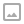## 「CF990G」GCD Counting-并查集/点分治

·  ✏️ About  1227 words  ·  ☕ 3 mins read · 👀... views

Codeforces 990G

## 代码

  1 2 3 4 5 6 7 8 9 10 11 12 13 14 15 16 17 18 19 20 21 22 23 24 25 26 27 28 29 30 31 32 33 34 35 36 37 38 39 40 41 42 43 44 45 46 47 48 49 50 51 52 53 54 55 56 57 58 59 60 61 62 63 64 65 66 67 68 69 70 71 72 73 74 75  #include using namespace std; typedef long long ll; const int MAXN = 300000; int gcd(int x,int y){ return y == 0?x:gcd(y,x%y); } namespace BCJ{ int f[MAXN],siz[MAXN]; int find(int x){ return f[x] == x?x:f[x] = find(f[x]); } void un(int x,int y,ll &cur){ int fx = find(x),fy = find(y); if(fx == fy) return; cur -= 1LL*siz[fx]*(siz[fx]+1)/2; cur -= 1LL*siz[fy]*(siz[fy]+1)/2; f[fy] = fx,siz[fx] += siz[fy]; cur += 1LL*siz[fx]*(siz[fx]+1)/2; } } struct Edge{ int u,v; }edge[MAXN]; vector V[MAXN]; vector E[MAXN]; int n,a[MAXN]; void init(){ scanf("%d",&n); for(int i = 1;i<=n;i++){ scanf("%d",&a[i]); V[a[i]].push_back(i); } for(int i = 1;i<=n-1;i++){ scanf("%d %d",&edge[i].u,&edge[i].v); E[gcd(a[edge[i].u],a[edge[i].v])].push_back(i); } } void solve(){ int maxn = 200000; static ll res[MAXN],cur = 0; for(int i = maxn;i>=1;--i){ cur = 0; for(int j = i;j<=maxn;j+=i){ for(int k = 0;k i) res[i] -= res[j]; } for(int j = i;j<=maxn;j+=i) for(int k = 0;k

  1 2 3 4 5 6 7 8 9 10 11 12 13 14 15 16 17 18 19 20 21 22 23 24 25 26 27 28 29 30 31 32 33 34 35 36  // 非本人创作 #include #define gcd(a,b) __gcd(a,b) using namespace std;const int N=2e5+7;typedef long long ll; struct data{int to,next;}e[N<<1];int n,m,i,j,cnt,a[N],d[N],vis[N],head[N],f[N],q[N],qq[N],u,v,root,sum,num[N],Tnum[N],size[N],fr[N],T,tt,tot;ll ans[N]; void ins(int u,int v){e[++cnt].to=v;e[cnt].next=head[u];head[u]=cnt;} void insert(int u,int v){ins(u,v);ins(v,u);} void getroot(int x,int fa){ size[x]=1;f[x]=0; for(int i=head[x];i;i=e[i].next)if(e[i].to!=fa&&!vis[e[i].to]) getroot(e[i].to,x),size[x]+=size[e[i].to],f[x]=max(f[x],size[e[i].to]); f[x]=max(f[x],sum-size[x]); if(f[x]WRITTEN BY
cqqqwq
A student in Computer Science.